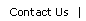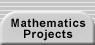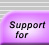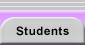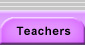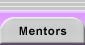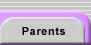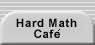# The Geometry of Complex Numbers

Any nonzero complex number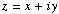can be described in polar form in terms of its modulus and argument.   If you plot z in the complex plane (where the x axis is the real part and the y axis is the imaginary part) at, then the modulus of z is the distance, r, from the origin to P.  The argument of z is the angle,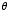, that the ray OP makes with the positive real axis.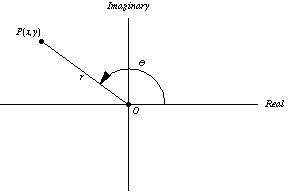Using basic trigonometry, we can write z as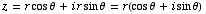This last expression is often abbreviated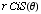.

You might notice that zero, which is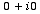, has a modulus zero, but has no unique argument.  Since our main reason for considering this form of complex numbers is for multiplying them, this deficiency of zero won't really be an issue.

Now if you multiply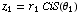and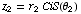and expand this messy expression, you will see some patterns that might be familiar.  In the last step we apply the angle sum formulae for sine and cosine.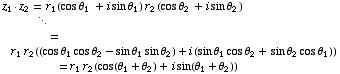The Rule to Remember:  When you multiply complex numbers, you multiply moduli and add arguments.

This is actually the way many mathematicians remember the trig identities.  Once you know this general rule for multiplying complex numbers, you don't need to memorize the details of the two identities we used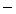you can always reconstruct them by knowing that the last step above is true!

 De Moivre's formula, named after Abraham de Moivre (1667 - 1754), follows from this more general rule.  Given any nonzero complex number z and any integer n, thepower of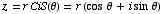is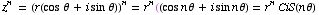Related Links: Dave's Short Course on Complex Numbers provides a thorough and accessible introduction to complex numbers, their meaning, geometry, and operations. Geometry and Complex Numbers offers a text with exercises on complex numbers and trigonometry. Complex Applet provides an interactive tool for studying the geometry of complex numbers. John and Betty's Journey Through Complex Numbers is a picture book introduction to complex numbers.Translations of mathematical formulas for web display were created by tex4ht. © Copyright 2003 Education Development Center, Inc. (EDC)